# Maths Brain Teasers 122 :: Can You - Guess The Next Number???Hey All; @StemGeeks Mathematician;

Without further, ado, let's get to our puzzle for today:: Maths Brain Teasers 122 :: Can You - Guess The Next Number???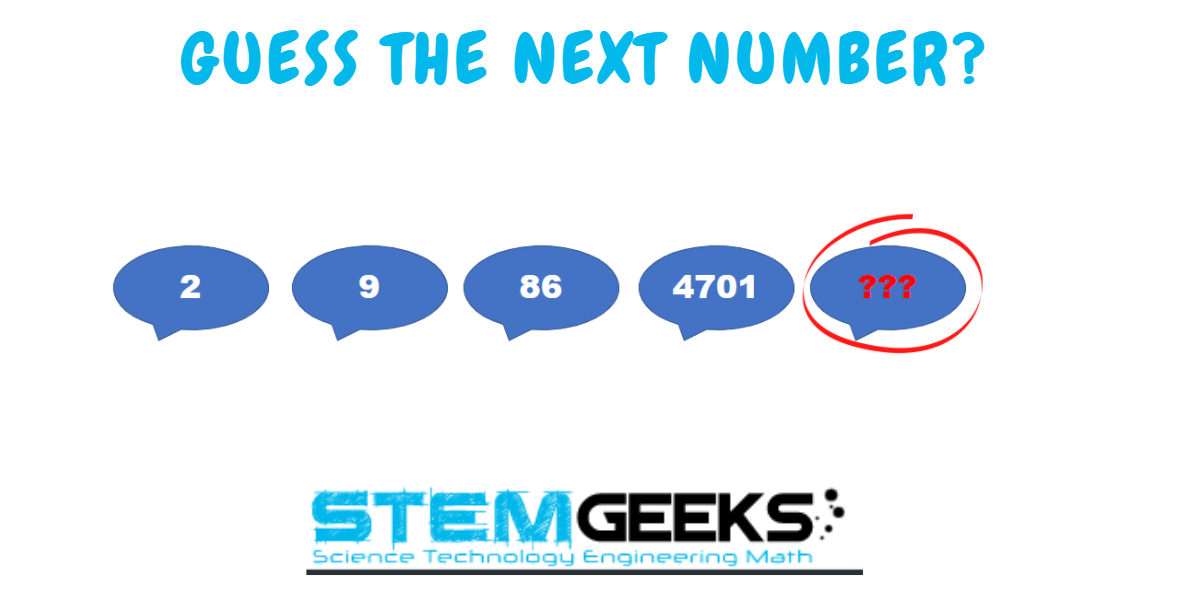Have a close look at the image given and see if you can find the correct number that replaces the question mark.

There is logic to resolve the puzzle; try finding out the logic and it should be resolved in seconds.. Guesswork isn't going to help...

With that, I'll leave you all with the Maths Brain Teasers 122 :: Can You - Guess The Next Number??? Good luck solving the puzzle...

##### Maths Brain Teasers 121 :: Observe the Pattern & Solve the Puzzle??? - Solved with Explanation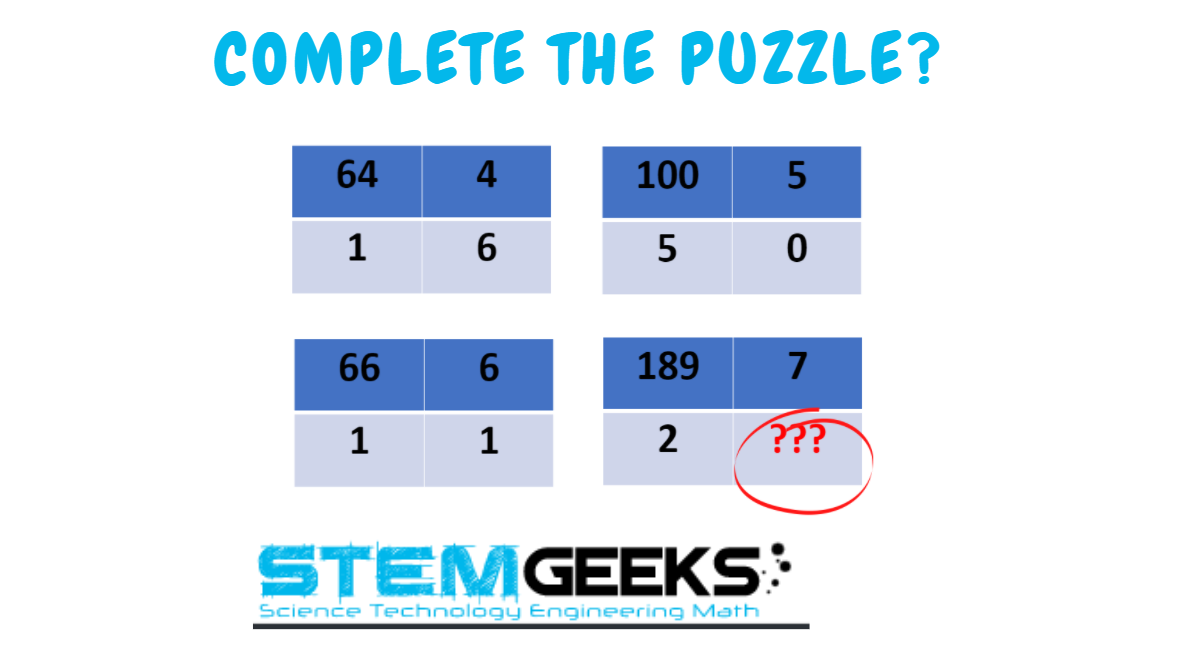Let's have a look at the Step by Step instructions to Solve the Maths Brain Teasers 121 :: Observe the Pattern & Solve the Puzzle???

If you carefully look at the Image in question; You would have noticed the following pattern is being followed::

### First-row first number divide by First row second number i.e.:: 64/4 = 16

One and Six add to the second row as separate numbers...

Let's take the image in question now::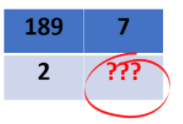### 189/7 = 27

Number 2 comes as the second-row first element and then number seven as second-row second element...

The way how @serhotest has solved it is also exceptional::

Yes, you are right there was a mistake in the image::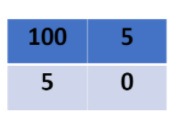### 5 should have been replaced with 2; :-) my bad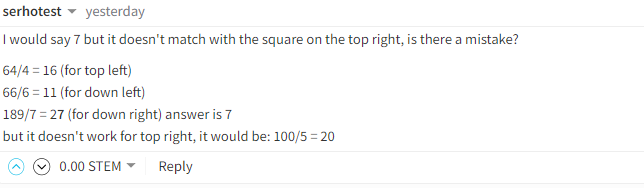Finally, the answer to the puzzle is Number:: 7

### STEM token GiveAway

I'll be again doing a giveaway of STEM tokens to the lucky random winner with the correct answer. For the last contest, which was Maths Brain Teasers 121 :: Observe the Pattern & Solve the Puzzle???.

We had 2 entries and I am happy to see the engagement. At the same time, it's great to see the detailed answers provided by everyone as to how they arrived at the solution of the puzzle.. Well done guys.. Way to GO... Now the results part; all the entries provided the right answer. So I am going to use a random comment picker tool to pick one Winner. And the Winner is::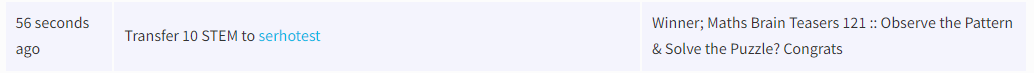Congratulations @serhotest - You WIN yourself 10 STEM tokens. You should be having the rewards in your STEM Wallet Soon.

### Math Quote for the Day::

Here is the motivation to solve the Maths Equation Puzzle?If you like my work, then please spread the Word.. that we do have the Math Brain Teasers competition here @StemGeeks platform. Reblog is much appreciated.

Best RegardsFind Me on the Other Social Media Platforms::PS:: All the Maths Brain Teasers; are made by me using the Pro Canva License Version

0
0
0.000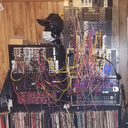Ha I was wondering what was up with the last one too

0
0
0.000(Edited)

Ok here's my theory on this one ... It's another typo and the 4701 is actually 7401 and the answer is 54,774,806

Thus

2x2 +5=9
9×9 +5= 86
86 x 86+5= 7401

Thus 7401×7401+5=54,774,806

Eh, it's worth a shot

0
0
0.0009 = 2x2 +5
86 = 9x9 + 5
7401 = 86x86 + 5
Next should be 7401x7041+5 = 54774806

But as per question There is 4701 so
With same logic application

4701x4701+5= 22099406

0
0
0.000